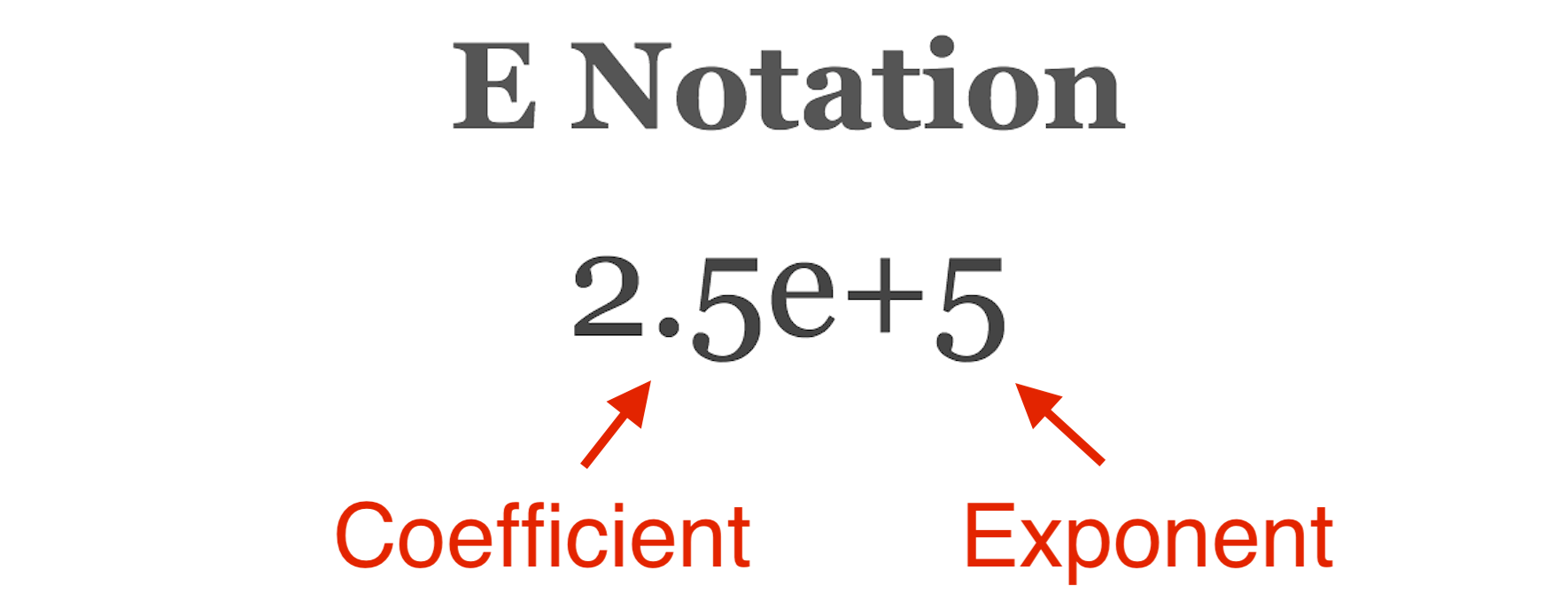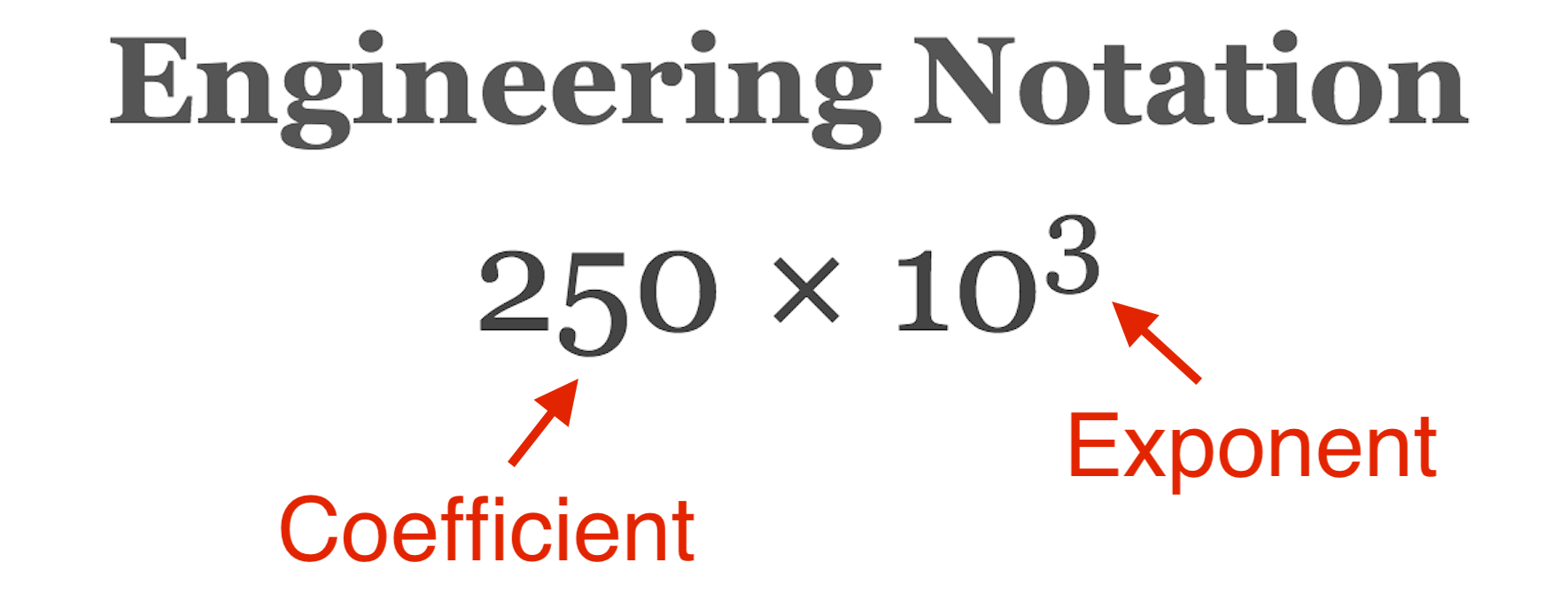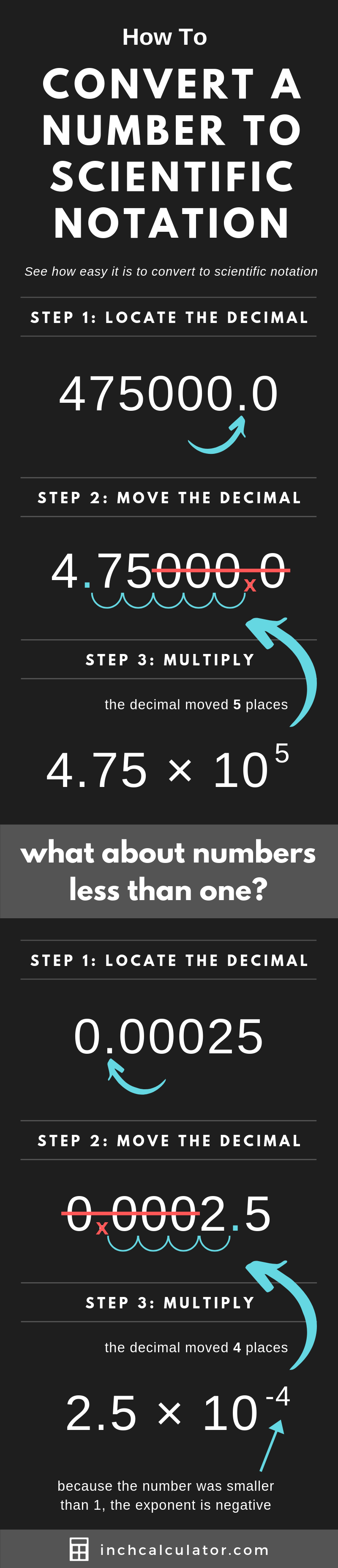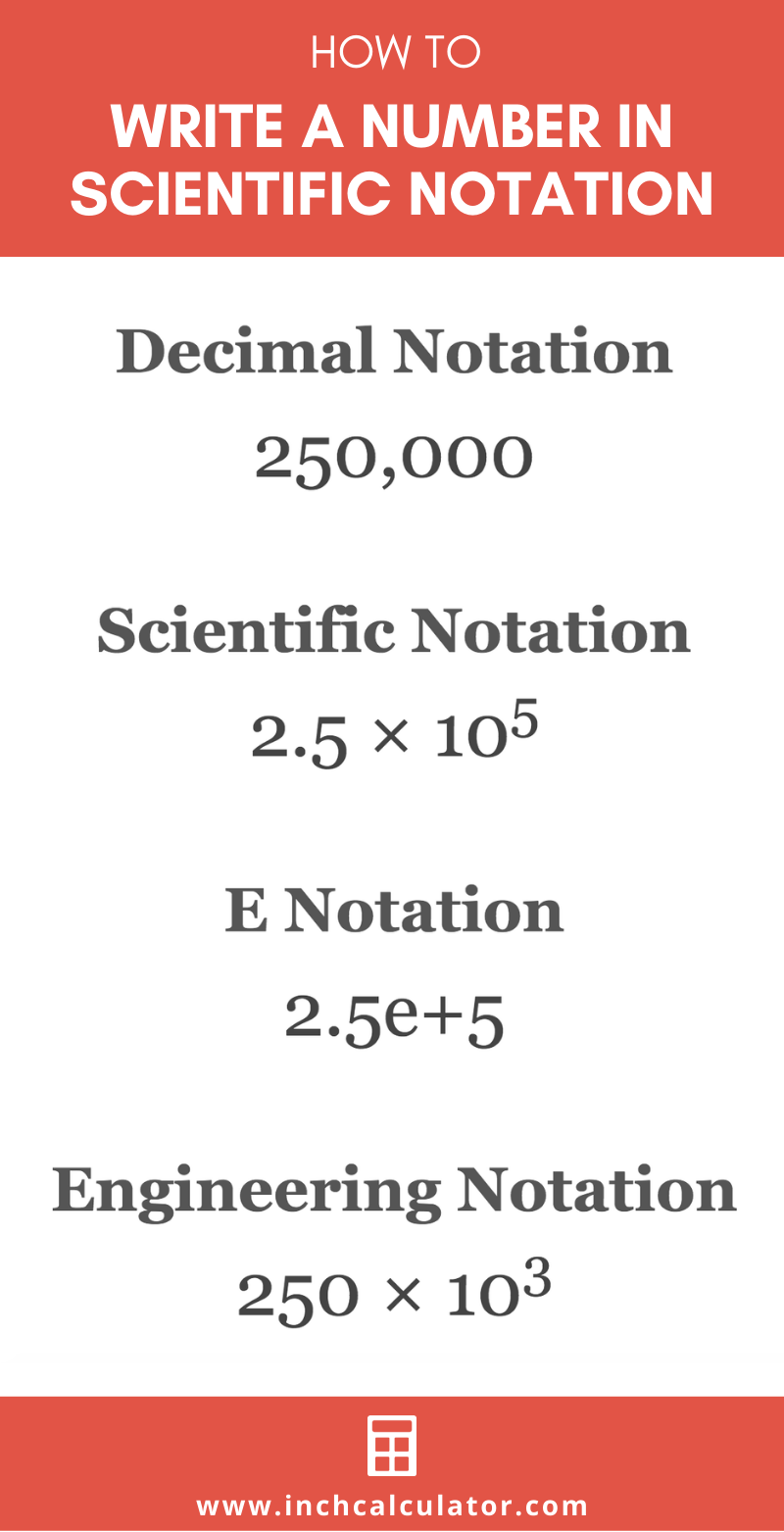# Scientific Notation Calculator and Converter

Convert a number to scientific notation, E notation, or engineering notation or vice-versa using the scientific notation calculator.

Hint: use either 5.2e+8 or 5.2 × 10^8 form to represent scientific notation.

## Solution:

5.2 × 108

5.2e+8

520 × 106

### Decimal Notation

520000000.0
Learn how we calculated this below

## How to Convert Scientific Notation to Decimal Form

Scientific notation, also called standard form, represents very large numbers or very small numbers in a shorter format. Numbers in scientific notation are an equation of a coefficient multiplied by 10 to the nth power.Here’s an example:

1.5 × 102

The number to the left of the multiplication symbol is the coefficient, or sometimes also called the mantissa. These are the significant digits in the number.

The number of digits in the coefficient will vary depending on how many significant figures are in the number. Use our significant figures calculator to find significant figures or round a number to prepare for a conversion to scientific notation.

### Convert Using a Calculator

To convert a number that is currently in scientific notation to decimal notation, simply solve the equation. To solve, multiply the coefficient by ten to the nth power.

You can also use our exponent calculator to solve the second part of the equation, then multiply the result by the coefficient.

For example, let’s convert 1.5 × 102 to decimal form.

1.5 × 102 = 1.5 × 100
1.5 × 102 = 150

### Convert Scientific Notation without a Calculator

We’ve shown you how to convert a number expressed in scientific notation to a decimal by solving, but this would get more difficult to do manually as the exponent gets larger. There’s an alternate way to convert to decimal without solving the equation.

If the exponent is positive, move the decimal point in the coefficient to the right one space for each value in the exponent.

For instance, if the exponent is two, then move the decimal point to the right two spaces. If the exponent is negative, then move the decimal point to the left.

For example, let’s convert 1.5 × 102 to a decimal using this method.

1.5 × 102 = 15.0 × 101
1.5 × 102 = 150.0 × 100
1.5 × 102 = 150.0

## How to Convert a Decimal to Scientific Notation

Converting a decimal number to scientific notation can be done in a few simple steps.

### Step One: Create Initial Equation

First, create an equation with the initial decimal being the coefficient multiplied by 10 to the power of 0.

For example, let’s create the starting scientific notation equation for the decimal 475,000.
475,000 = 475,000 × 100

### Step Two: Move the Decimal Point

Now that we have an equation, the second step is to move the decimal point in the coefficient until there is one significant digit to the left of the decimal point. For each space the decimal point is moved to the left, increase the exponent by 1.

Continuing the example above, move the decimal point to the left and increment the exponent.

475,000 = 475,000.0 × 100
475,000 = 47,500.0 × 101
475,000 = 4,750.0 × 102
475,000 = 475.0 × 103
475,000 = 47.5 × 104
475,000 = 4.75 × 105

### What About Numbers Less Than One?

The method above works for large numbers, but the method for handling small numbers less than one is a bit different.

Instead of moving the decimal point to the left in the coefficient, the decimal point needs to be moved to the right until one non-zero significant digit is to the left of the decimal point. Because the number is getting larger, we need to decrement a number from the exponent every time the decimal point is moved.

For example, let’s convert 0.00025 to scientific notation.

0.00025 = 0.00025 × 100
0.00025 = 0.0025 × 10-1
0.00025 = 0.025 × 10-2
0.00025 = 0.25 × 10-3
0.00025 = 2.5 × 10-4

## How to Use Exponential Notation

Exponential notation, or E notation, is another form of scientific notation where the “× 10” part of the equation is replaced with an “e+”.

The “E” in E notation stands for exponential. This form is also commonly referred to as scientific E notation.For example, let’s represent 1.5 × 102 in E notation.
1.5 × 102 = 1.5e+2

## How to Convert to Engineering Notation

Not to be confused with E notation, engineering notation is an alternate form of scientific notation where the exponent is a multiple of 3.

To convert scientific notation to engineering notation, move the decimal point in the coefficient to the right and reduce the exponent until the exponent is a multiple of 3.For example, let’s represent 1.5 × 105 in E notation.
1.5 × 105 = 15.0 × 104
1.5 × 105 = 150.0 × 103

## Scientific Notation and Decimal Equivalents

Decimal numbers and the equivalent values in scientific notation, E notation, and engineering notation.
Decimal Number Scientific Notation E Notation Engineering Notation
1 1 × 100 1E+0 1 × 100
1,000 1 × 103 1E+3 1 × 103
72,000 7.2 × 104 7.2E+4 72 × 103
1,349.12 1.34912 × 103 1.34912E+3 1.34912 × 103
0.000000435 4.35 × 10-7 4.35E-7 435 × 10-9
-7,500 -7.5 × 103 -7.5E+3 -7.5 × 103
-0.00005234 -5.234 × 10-5 -5.234E-5 -52.34 × 10-6

## Visual Guide to Convert to Scientific Notation

The infographic below demonstrates visually how to convert a number to scientific notation.